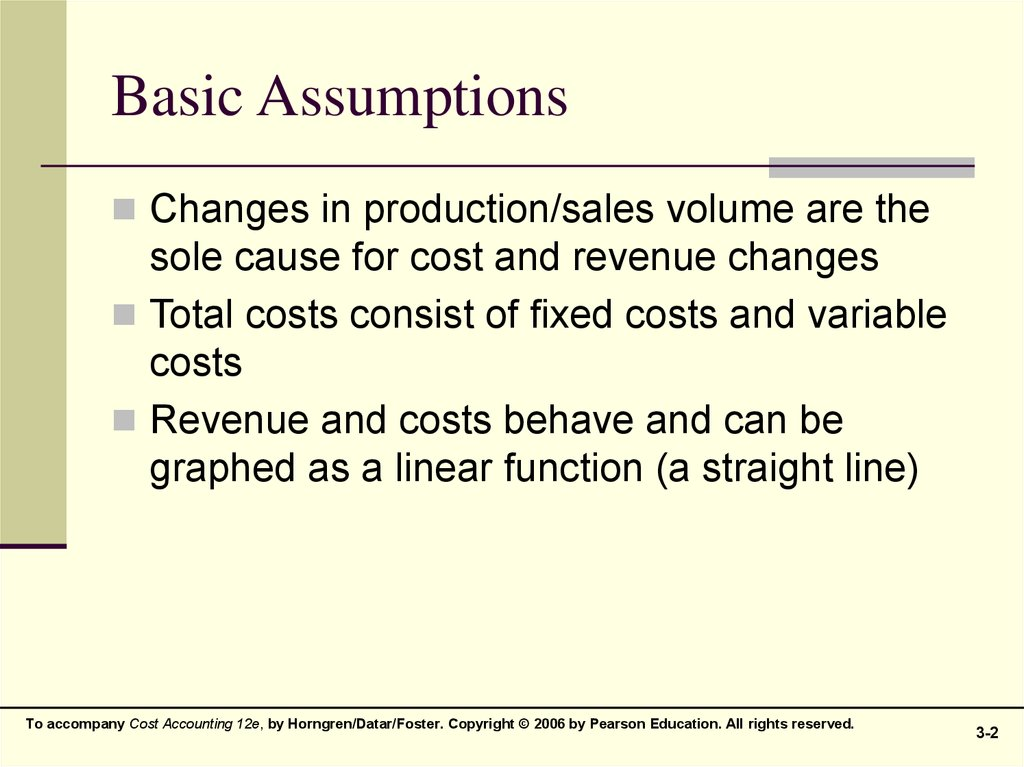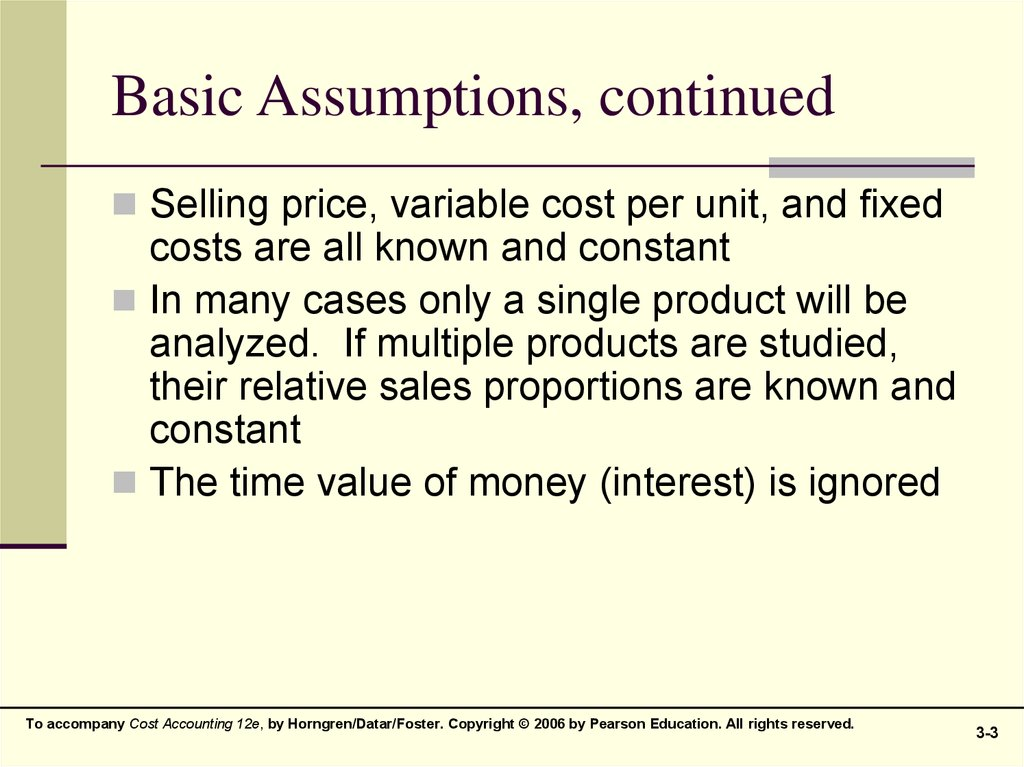# Cost-Volume-Profit (CVP) Analysis

## 1. CHAPTER 3

Cost-Volume-Profit
(CVP)
Analysis

## 2. Basic Assumptions

Changes in production/sales volume are the
sole cause for cost and revenue changes
Total costs consist of fixed costs and variable
costs
Revenue and costs behave and can be
graphed as a linear function (a straight line)
3-2

## 3. Basic Assumptions, continued

Selling price, variable cost per unit, and fixed
costs are all known and constant
In many cases only a single product will be
analyzed. If multiple products are studied,
their relative sales proportions are known and
constant
The time value of money (interest) is ignored
3-3

Operating
Income
=
Net
Income
Total
Revenues
from
Operations
=
Operating
Income
Cost
of
Goods
Sold
Pretax
Operating
Expenses
Income
Taxes
3-4

## 5. Contribution Margin

Contribution Margin equals sales less
variable costs
CM = S – VC
Contribution Margin per unit equals unit
selling price less variable cost per unit
CMu = SP – VCu
3-5

## 6. Contribution Margin

Contribution Margin also equals contribution
margin per unit multiplied by the number of
units sold
CM = CMu x Q
Contribution Margin Ratio (percentage)
equals contribution margin per unit divided by
selling price
CMR = CMu ÷ SP
3-6

## 7. Contribution Margin Income Statement Derivations

A horizontal presentation of the Contribution
Margin Income Statement:
Sales – VC – FC = Operating Income (OI)
(SP x Q) – (VCu x Q) – FC = OI
Q (SP – VCu) – FC = OI
Q (CMu) – FC = OI
Remember this last equation, it will be used
again in a moment
3-7

## 8. CVP, Graphically

\$10,000
y
Operating
income
Total
revenues
line
\$8,000
Breakeven point = 25 units
Operating
income area
Dollars
\$6,000
\$5,000
Total
costs
line
Variable
costs
Breakeven
point
= 25 units
\$4,000
Total
costs
line
\$2,000
Operating
loss area
Operating
loss area
x
10
20
25
30
40
Fixed
costs
50
Units Sold
3-8

## 9. Breakeven Point

Recall the last equation in an earlier slide:
Q (CMu) – FC = OI
A simple manipulation of this formula, and
setting OI to zero will result in the Breakeven
Point (quantity):
BEQ = FC ÷ CMu
At this point, a firm has no profit or loss at
a given sales level
3-9

## 10. Breakeven Point, continued

If per-unit values are not available, the
Breakeven Point may be restated in its
alternate format:
BE Sales = FC ÷ CMR
3-10

## 11. Breakeven Point, extended: Profit Planning

With a simple adjustment, the Breakeven
Point formula can be modified to become a
Profit Planning tool
Profit is now reinstated to the BE formula,
changing it to a simple sales volume equation
Q = (FC + OI)
CM
3-11

## 12. CVP and Income Taxes

From time to time it is necessary to move back and forth
between pre-tax profit (OI) and after-tax profit (NI),
depending on the facts presented
After-tax profit can be calculated by:
OI x (1-Tax Rate) = NI
NI can substitute into the profit planning equation
through this form:
OI = I I
NI
I
(1-Tax Rate)
3-12

## 13. Sensitivity Analysis

CVP provides structure to answer a variety of
“what-if” scenarios
“What” happens to profit “if”:
Selling price changes
Volume changes
Cost structure changes
Variable cost per unit changes
Fixed cost changes
3-13

## 14. Margin of Safety

One indicator of risk, the Margin of Safety
(MOS) measures the distance between
budgeted sales and breakeven sales:
MOS = Budgeted Sales – BE Sales
The MOS Ratio removes the firm’s size from
the output, and expresses itself in the form of
a percentage:
MOS Ratio = MOS ÷ Budgeted Sales
3-14

## 15. Operating Leverage

Operating Leverage (OL) is the effect that fixed
costs have on changes in operating income as
changes occur in units sold, expressed as
changes in contribution margin
OL = Contribution Margin
Operating Income
Notice these two items are identical, except for
fixed costs
3-15

## 16. Effects of Sales-Mix on CVP

The formulae presented to this point have assumed a
single product is produced and sold
A more realistic scenario involves multiple products
sold, in different volumes, with different costs
For simplicity’s sake, only two products will be
presented, but this could easily be extended to even
more products
3-16

## 17. Effects of Sales-Mix on CVP

A weighted-average CM must be calculated (in this
case, for two products)
Weighted ( Product #1 CMu x Product #1 Q ) + ( Product #2 CMu x Product #2 Q )
Average =
CMu
Total Units Sold (Q) for Both Products
This new CM would be used in CVP equations
Multi-
Fixed Costs
Product = Weighted Average CM per unit
BE
3-17

## 18. Multiple Cost Drivers

Variable costs may arise from multiple cost
drivers or activities. A separate variable cost
needs to be calculated for each driver.
Examples include:
Customer or patient count
Passenger miles
Patient days
Student credit-hours
3-18

## 19. Contribution Margin vs. Gross Profit Comparative Statements

Contribution Margin Income Statement
(Internal-Use Only)
Revenues:
Less:
Variable Cost of Goods Sold
Variable Operating Costs
Contribution Margin
Fixed Operating Costs
Operating Income
Financial Accounting Income Statement
GAAP - Based
\$200
\$120
45
165
35
20
\$15
Revenues:
Less:
Cost of Goods Sold
\$200
\$120
Gross Margin (Profit)
Fixed & Variable Operating Costs
Operating Income